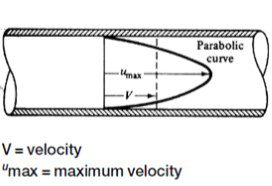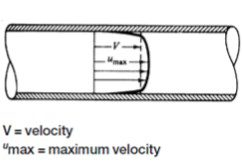At Holland, we are sizing and specifying sanitary pumps every day. Regardless of pump technology, the most ubiquitous piece of information when selecting a pump is flow rate. When selecting a line size or sizing a CIP pump, we look at flow velocity through the tube. So what is the difference you ask? This post will cover the difference between flow rate and flow velocity and how we use this to make proper pump selection.

To begin, the terms velocity and flow are confusing and are sometimes interchanged- this is a bad habit this post if going to try and help you break. Velocity is the distance a fluid moves with respect to time. In the case of a sanitary fluid flowing through a tube, velocity is often expressed in units of feet per second. Flow is volume of liquid or gas that is moving per unit of time. For a sanitary fluid moving in a piece of tubing, this is often expressed in gallons per minute. Flow and velocity can be related by the following equation:

Q= V*A

Where Q is flow rate, V is fluid velocity and A is the cross sectional area of the tubing. What does this equation tells us? It tells us that assuming line diameter is held constant, as velocity increases, flow will increase. It also tells us that 10 gallons per minute through a 1” line and 10 gallons per minute through a two inch line yield very different velocities (the velocity in the 1” line is about 4 times greater than that in the 2” line”).

So why is this important? Well, while most pumps are sized based on capacity, or flow rate, little consideration is given to line size and velocity. Flow through a tube can be either laminar or turbulent. The type of flow is often based on the Reynolds number (Re), with Re’s less than 2300 being considered “laminar” and Re’s greater than 4000 being considered turbulent. The Reynolds number can be calculated by the following equation:

Re= (Tube diameter (in) x Velocity (ft/s) x Density (lb/gallon)/Viscosity

Or

Re= 3162 x Flow (GPM)/Tube diameter (inches) x ViscosityWhat the Reynolds number ultimately tells us is that as our line size changes, our Reynolds number will change as well. The smaller the tube, the higher the Reynolds number (assuming a fixed capacity). When the Reynolds number is low, and flow is laminar, the fluid is moving through the tube in concentric layers, in a parabolic shape with the maximum velocity occurring at the center of the pipe and decreasing to zero at the pipe wall. Low velocity at the pipe wall creates very little friction.

In contrast to laminar flow, turbulent flow (high Reynolds number) has a flatter profile, with considerable mixing and scouring occurring at the tube wall, increasing frictional forces. The Reynolds number equation tells us that turbulent flows will occur with fluids with a high velocity and low viscosity.The frictional forces of turbulent flow are great when we want to create a scouring action at the line’s sidewalls for CIP, but detrimental when trying to minimize energy losses (i.e. long transfers with small pump motors). This is why we recommend using dedicated CIP supply pumps when cleaning to overcome the friction losses required, instead of relying on process pumps to do so.

To conclude, hopefully this posts sheds some light on the difference between flow rate and flow velocity. While flow rate and flow velocity are related, they are not the same, and both need to be taken into account when sizing a pump. With any questions about flow rate, flow velocity, or sizing a pump for your next application, contact a Holland Sales Engineer today.